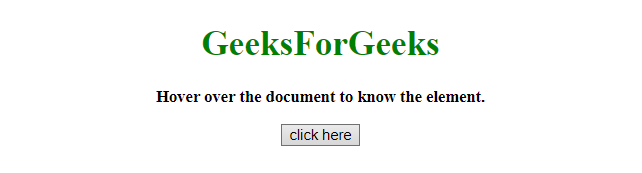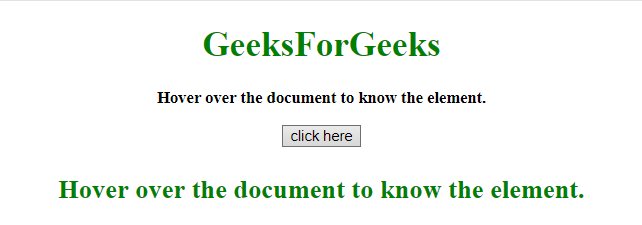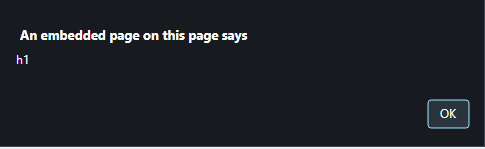Related Articles
How to determine which element the mouse pointer move over using JavaScript ?
• Last Updated : 12 Sep, 2019

Given an HTML document and the task is to get the element where mouse pointer moves over. There are two approaches to solve this problem which are discussed below:

Approach 1:

• Get the x and y coordinates value by using .clientX and .clientY property.
• Use document.elementFromPoint(x, y) method to get the element content on that position when mouse pointer moves over.

Example 1: This example implements the above approach.

 `  ` `<``html``>  ` ` `  `<``head``>  ` `    ``<``title``>  ` `        ``How to determine which element the mouse ` `        ``pointer move over using JavaScript ? ` `    ``  ` `  ` ` `  `<``body` `style` `= ``"text-align:center;"``>  ` `     `  `    ``<``h1` `style` `= ``"color:green;"` `onmouseover` `= ``"GFG_Fun()"``>  ` `        ``GeeksForGeeks  ` `    ``  ` `     `  `    ``<``p` `id` `= ``"GFG_UP"` `onmouseover` `= ``"GFG_Fun()"` `            ``style` `= ``"font-size: 15px; font-weight: bold;"``>  ` `    `` ` `     `  `    ``<``button` `onmouseover` `= ``"GFG_Fun()"``>  ` `        ``click here  ` `    ``  ` `     `  `    ``<``p` `id` `= ``"GFG_DOWN"` `style` `=  ` `        ``"font-size: 24px; font-weight: bold; color: green;"``>  ` `    `` ` `     `  `    ``<``script``>  ` `        ``var up = document.getElementById('GFG_UP');  ` `        ``var down = document.getElementById('GFG_DOWN');  ` `        ``up.innerHTML = "Hover over the document to know the element.";  ` `         `  `        ``function GFG_Fun() {  ` `            ``var x = event.clientX; ` `            ``var y = event.clientY; ` `            ``el = document.elementFromPoint(x, y); ` `            ``down.innerHTML = el.innerHTML; ` `        ``}  ` `    ``  ` ` ` ` `  ` `

Output:

• Before Hover on the button:• After Hover on the button:Approach 2:

• Attach the event ‘onmouseover’ to the element.
• Call the alert function with the id of that element, each time when event occurs.

Example 2: This example using the approach discussed above.

 `  ` `<``html``>  ` ` `  `<``head``>  ` `    ``<``title``>  ` `        ``How to determine which element the mouse ` `        ``pointer move over using JavaScript ? ` `    ``  ` `  ` ` `  `<``body` `style` `= ``"text-align:center;"``>  ` `     `  `    ``<``h1` `id` `= ``"h1"` `style` `= ``"color:green;"` `            ``onmouseover` `= ``"alert(this.id)"``>  ` `        ``GeeksForGeeks  ` `    ``  ` `     `  `    ``<``p` `id` `= ``"p"` `onmouseover` `= ``"alert(this.id)"` `            ``style` `= ``"font-size: 15px; font-weight: bold;"``>  ` `        ``Hover over the document to know the element. ` `    `` ` `     `  `    ``<``button` `id` `= ``"button"` `onmouseover` `= ``"alert(this.id)"``>  ` `        ``click here  ` `    ``  ` `  ` ` `  ` `

Output:

• Before Hover on the button:• After Hover on the button:My Personal Notes arrow_drop_up
Recommended Articles
Page :